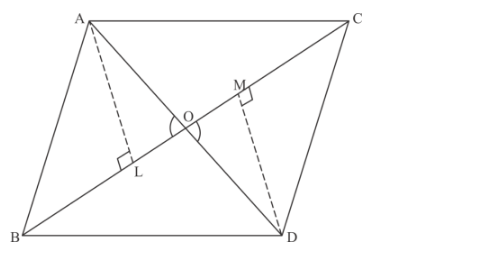# In the given figure, ∆ABC and ∆DBC are on the same base BC.`
Question:

In the given figure, ∆ABC and ∆DBC are on the same base BC. If AD and BC intersect at O, prove that Area∆ABCArea∆DBC=AODO.Solution:

Given: ΔABC and ΔDBC are on the same base BC. AD and BC intersect at O.

Prove that: $\frac{\operatorname{Ar}(\triangle \mathrm{ABC})}{\operatorname{Ar}(\triangle \mathrm{DBC})}=\frac{\mathrm{AO}}{\mathrm{DO}}$

Construction: Draw $\mathrm{AL} \perp \mathrm{BC}$ and $\mathrm{DM} \perp \mathrm{BC}$.

Now, in ΔALO and ΔDMO, we have

$\angle \mathrm{ALO}=\angle \mathrm{DMO}=90^{\circ}$

$\angle \mathrm{AOL}=\angle \mathrm{DOM}$ (vertically opposite angles)

Therefore $\triangle \mathrm{ALO} \sim \triangle \mathrm{DMO}$

$\therefore \mathrm{ALDM}=\mathrm{AODO}$ (Corresponding sides are proportional)

Ar∆ABCAr∆BCD=12×BC×AL12×BC×DM                       =ALDM                       =AODO